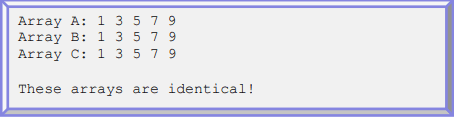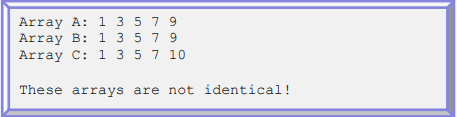# Trouble with an array program in C

• dect117
In summary, the conversation discussed the task of writing a function to check if three arrays of integers are identical. Different approaches were suggested, including using for-loops, Boolean variables, and matrix comparison methods. It was also recommended to hardcode some array values or take them from a file for testing purposes. Additionally, it was advised to set up programs for testing in order to save time.

## Homework Statement

Write a function that finds if three arrays of integers are identical. The arrays are identical when they have the same values at the same indices. Below are two samples outputs.N/A

## The Attempt at a Solution

I decided to compare the arrays using a for-loop. I feel like that's a messy way to go about it but I'm not really sure how else I can make it work. Here's my code.
Code:
#include <stdio.h>
#define SIZE 5
int main () {
int i, x, arr1[SIZE], arr2[SIZE], arr3[SIZE];
printf ("Array A: ");
for (i=0; i<SIZE; i++)
scanf ("%d", &arr1[i]);
printf ("Array B: ");
for (i=0; i<SIZE; i++)
scanf ("%d", &arr2[i]);
printf ("Array C: ");
for (i=0; i<SIZE; i++)
scanf ("%d", &arr3[i]);
for (i=0; i<SIZE; i++){
if (arr1[i]==arr2[i] && arr2[i]==arr3[i]) {
x==1;
}
else if(!(arr1[i]==arr2[i] && arr2[i]==arr3[i])) {
x==2;
break;
}
}
if (x==1)
printf ("\nThese arrays are identical!");
else if (x==2)
printf ("\nThese arrays are not identical!");
return 0;
}
It prints "These arrays are identical!" regardless of whether or not that is true. I'm not understanding why it won't work.

#### Attachments

The statements x==1 and x==2 should be x=1 and x=2.

Also:
the "else if" part is not needed. It can be a simple "else".

I would have declared:
Code:
bool bDifferenceFound;

Then before the comparison loop, I would say:
Code:
bDifferenceFound = false;

Then in the loop:
Code:
  bDifferenceFound = (arr1 != arr2) || (arr2 != arr3);
if(bDifferenceFound) break;

Last edited:
Look at your syntax for the assignment operators...

Wow, thanks a lot guys! I have to pay more attention.

Since your Question 3 requested alternate forms:
Consider using Boolean variables and tests to simplify logic statements as detailed in response #2.

Since you wrote a "For" loop to input the values into arrays, it seems natural to use more For loops to evaluate the arrays but consider other iteration and matrix comparison methods since your code locates the first mismatch, sets Boolean "false", then dumps the loop.

If your math includes matrix algebra, consider methods to determine if Mat_A = Mat_B for values contained in same dimension matrices.
(Forgive me if I exceed the boundaries of your thread as I'm new to answering homework questions online. --Norm)

It would be easier to hardcode some array values or take them from a file while testing your program - and faster since you won't have to insert values.
Then change it back later.
You could also just check if they are not the same as suggested earlier - and if you never hit that break then they ought to be the same right?
Know I'm a little late but wanted to give some advice for future assignments - setting up programs for testing will save lots and lots of time.